# Predicting Primes

## Lambda School Unit 2 Portfolio Project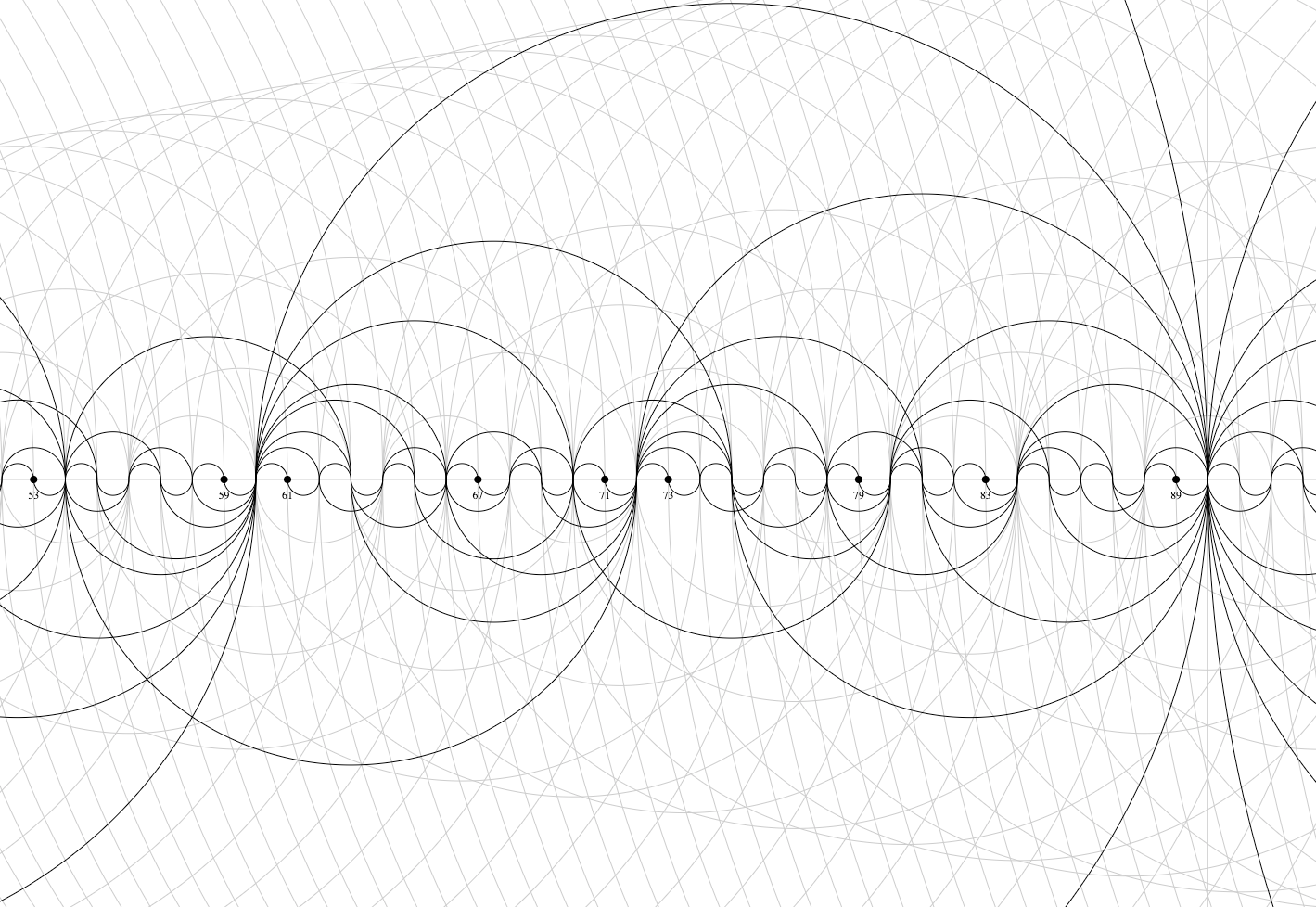Image source: Jason Davies

## What is a prime number?

• A prime number is a number with only two factors, itself and one.
• A prime number is only divisible by itself and one.
• A prime number cannot be produced by any two numbers (not including itself).

The first few primes are: {2, 3, 5, 7, 11, 13, 17, 19, 23, …}

Notice there are numbers missing in the above set if we expect to count in order (1, 2, 3, 4…). This is because all those missing numbers are some combination of the primes. So we depend on primes to make up all the other numbers we use for anything that we might measure with a number.

## Why do we care?

“It is evident that the primes are randomly distributed but, unfortunately, we don’t know what ‘random’ means” (Vaughan, 1990)

This has always baffled me! If we know how to figure out what numbers are primes, and the rest of the numbers are combinations of primes, then why is our understanding of their behavior limited to ‘random’?

We can always say something about something. Let’s see if we can say something more, something more insightful about the behavior of prime numbers. The second fact in the following quote is more aligned with our sentiment:

“There are two facts about the distribution of prime numbers which I hope to convince you so overwhelmingly that they will be permanently engraved in your hearts.

The first is that despite their simple definition and role as the building blocks of the natural numbers, the prime numbers… grow like weeds among the natural numbers, seeming to obey no other law than that of chance, and nobody can predict where the next one will sprout.

The second fact is even more astonishing, for it states just the opposite: that the prime numbers exhibit stunning regularity, that there are laws governing their behaviour, and that they obey these laws with almost military precision.”

D. Zagier from “The first 50 million prime numbers”, The Mathematical Intelligencer 0 (1977) 7

## Feature Engineering

A DataFrame is a matrix of columns (features) and rows (observations). Our columns will eventually be separated into an input matrix X (independent variables) and an output vector y (dependent variables).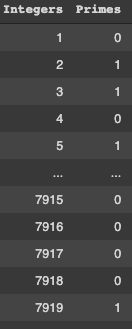Primes with binary classification

We want to predict primes, but we have primes as our only feature! We need features about primes that accurately describe the underlying structure of numbers.

How can we predict primes with primes?

It is a known fact about primes that for each prime p > 3, we will only ever find them in a 6k -1 or 6k +1 position, e.g., 17 and 43. Hence, we can say that a prime p is only ever on the left-hand side (LHS), or the right-hand side (RHS) of a multiple of 6.

So we will add two features: One LHS column, and one RHS column for each prime p in its respective position. The chart below shows primes up to 100, where 1 = prime. We readily see what we mean by LHS and RHS primes: the orange dots correspond to LHS primes, and the blue dots correspond to RHS primes.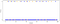1 = Prime (up to 100 (Orange Dot: LHS primes. Blue Dot: RHS primes))

Below, a line represents a LHS prime. From this chart we get a sense of how dense these primes are within this range up to the 1000th prime (7919).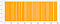LHS primes within the first 1000 primes

RHS primes also appear to be quite dense in this range: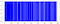RHS primes within the first 1000 primes

Now we have two features in our integer DataFrame:

The first 1000 primes split into:

• Left-hand side primes
• Right-hand side primes

## Predictive Model Building

A standard way of splitting data is 80% on the test set, 10% on the validation set and the remaining 10% on the test set which is held out from our model until the very end. We follow this guideline in building our model.

Since we want to predict the class our observations belong to (prime or not), let’s try a quick Logistic Regression model (classification model) to see what sort of predictions we can make with our current information. We will let that inform whether we should add more information about primes as additional features.

## Check Metrics

Take the absolute value of prediction error (convert all values to positive) and then calculate the mean for all absolute error values. Below is our first logistic regression model accuracy scores: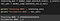Mean Absolute Error

A pleasant surprise!

Assuming this model does not actually accurately predict primes 100% of the time, let’s introduce some more features. But what to add? We want to predict primes, so we have to assume this is a dependent variable. What do primes depend on? We need an interpretation of primes that offers additional meaningful information that we can encode into our model. We are affirmed in our conviction when Leo Breiman says, “the point of a model is to get useful information about the relation between the response and predictor variables,” and “the goal is not interpretability, but accurate information” (Breiman, 2001).

## Back To The Drawing Board

Recall the fact about prime positions occupying 6𝑘±1 for each prime 𝑝>3. We call 6𝑘−1 a left-hand side position (LHS), being on the left of each 6𝑘 on a number line; and 6𝑘+1 a right-hand side position (RHS), being on the right of each 6𝑘. Observing those positions where there is not a prime, we see it is not prime because it is composite. That is, these positions are occupied by integers that are composed of at least two previous primes. This means any composite integer not divisible by 2 or 3 is composed of some combination of LHS primes and/or RHS primes. In fact, this is so because any 2𝑚 and 3𝑛 both divide some 6𝑘 and all other integers are “confined” to either position of 6𝑘±1. We note the first few in this collection: {25, 35, 49, 55,…}. These integers can be represented by their unique factorizations: {5⋅5, 5⋅7, 7⋅7, 5⋅11,…}.

Let us assume each of these positions 6𝑘±1 wants to be prime. We can say that a composite integer 𝑐 is not prime because at least two previous primes coincide at 𝑐. We interpret this occurrence as primes blocking a potential prime. For example: 25 is composed of two occurrences of 5. Then we interpret 25 as blocked from being prime by 5. Our second composite from our collection above is 35 and we say it is blocked from being prime by 5 and 7. This continues: 5 (and all further LHS, RHS primes) will block a potential prime for each of its multiples not divisible by 2 and/or 3.

Let us consider any prime 𝑝 as an occurrence, and each of its multiples 𝑝 ⋅ (2,3,4,5…)𝑟 as a recurrence. Then a composite in the form of 6𝑘±1 is not prime because of prime recurrences. Because both LHS and RHS primes block potential primes with each of their recurrences it is meaningful to keep track of these blockages. When studying primes the tendency seems to be focusing only on where a prime is, but never why a prime is. Asking, “why prime?” led us to see “why not prime.” Keeping an account of “why not prime” may help teach our model where a prime is.

Recall that we already have a column for LHS and RHS primes, and these are their occurrences. Now we will add additional features by adding a column of recurrences for each LHS and RHS prime.

Note, since we are only concerned about training our model, we need only account for prime recurrences for those primes that recur within the range of our training model. That is, we are not adding a column for all 1000 primes. We need only add a column for each prime that has, at least, its fifth multiple within our training set.

## Re-Engineer, Re-Split, Re-Build, Re-Train

“Random forests are an effective tool in prediction. Because of the Law of Large Numbers they do not overfit. Injecting the right kind of randomness makes them accurate classifiers and regressors. Furthermore, the framework in terms of strength of the individual predictors and their correlations gives insight into the ability of the random forest to predict. Using out-of-bag estimation makes concrete the otherwise theoretical values of strength and correlation.”

Breiman L: Random forests. J Mach Learn 2001, 45: 5–32. 10.1023/A:1010933404324

A Random Forest is an ensemble of Decision Trees. This model will make a prediction on each individual tree it produces, and then averages those predictions to predict a class that gets the most votes, e.g., prime or not. (Aurélien Géron).

## Check Metrics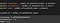Random Forest Classifier Prediction Score

Again we appear to be accomplishing the ideal!

## Check Specific Parameters for Hyperparameter Tuning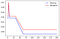Predictive results based on n_estimators (number of trees in forest)

At about 30 trees further increase in the number of trees decreases the validation performance.Predictive results based on max_depth (maximum depth of the tree)

At about 7–8 tree depth, our model plateaus at the highest prediction value. We want to constrain this parameter to regularize our model which helps reduce the risk of overfitting — since the deeper we let our tree grow, the more splits occur thus more is learned about the data.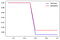Predictive results based on min_samples_splits (min number of samples required to split an internal node)

At about 50% our model does not learn enough about the data. That is, an increase in this parameter means more constraint for each tree in the forest as more samples are considered at each node. If we let this model use all of the samples at each node (1.0 on the x-axis) we would have a case of underfitting.

## Tuned Model Results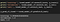Tuned Model Prediction Score

## Random Forest Feature Importance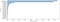Top 20 Feature Importances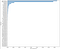Top 50 Feature Importances

One might assume that the gini feature importance is biased towards high-cardinality features: If this were the case in this model then the feature ‘5_blocks’ would have the highest importance as it has the highest cardinality — 19 higher than ‘LHS_Primes’. ‘5_blocks’ is nowhere close!

The importance is verified with a Permutation Feature Importance inspection.

“Permutation feature importance overcomes limitations of the impurity-based feature importance: they do not have a bias toward high-cardinality features and can be computed on a left-out test set.” 

“The permutation feature importance is defined to be the decrease in a model score when a single feature value is randomly shuffled. This procedure breaks the relationship between the feature and the target, thus the drop in the model score is indicative of how much the model depends on the feature” 

Since we randomly shuffled the values in the entire column, we expect the permuted set would change the accuracy given that these values in our feature columns are all position based and need to be where they are. Then the model accuracy going down is no surprise given the importance of this feature in our constructed model.

The absence of a significant drop in score reflects a lack of dependence on this particular feature. Although this feature has some importance, it is not enough relative to the dominant features of LHS and RHS columns. Even though ‘5_blocks’ has more values!

## INSIGHTS FROM MODEL

• Potential drawback: Our range is simply too small.
• Gini Importance metric: Why is 17 less important than 23 and 29? and 19 less important than 31 and 37? all of which recur less frequently than 17 and 19. Some RHS recurrences are more important than LHS recurrences, and vice versa. One might reasonably expect the recurrence columns to be in ordinal order. With more features shown, we see this does not hold at all! How to interpret?
• Initial thought: Encode all 1000 primes with their own recurrence columns. With a moments reflection we realize that the vast majority of the primes in the first 1000 prime range will not recur in the same range. For example: the 999th prime (7907) obviously does not have its second multiple before 7919. Since the first LHS/RHS recurrence begins to occur for each prime’s fifth multiple, we need only account for the first 1/5 of the primes in our range! This means, in our POV of primes being determined by previous primes blocking potential primes, that no matter how large our range is we need only account for the first 1/5 of the primes and their multiples in that range to determine the entirety of the primes within that range! This statement is formulated in the conjecture below:

## Conjecture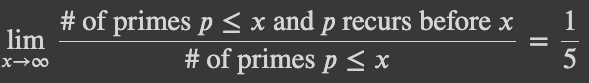where recurs means p reaches its 5th multiple before x, i.e., p blocks at least one potential prime position before x